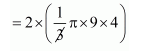# The height of a solid cylinder is 15 cm

Question:

The height of a solid cylinder is 15 cm and the diameter of its base is 7 cm. Two equal conical holes each of radius 3 cm, and height 4 cm are cut off. Find the volume of the remaining solid.

Solution:

Volume of cylinder

$=\pi r^{2} h$

$=\pi \times\left(\frac{7}{2}\right)^{2} \times 15$

$=\frac{735}{4} \pi \mathrm{cm}^{3}$

$=183.75 \pi \mathrm{cm}^{2}$

Volume of cones

$=2 \times\left(\frac{1}{3} \pi r^{2} h\right)$$=24 \pi \mathrm{cm}^{3}$

Volume of remaining solid

$=183.75 \pi-24 \pi$

$=502.1 \mathrm{~cm}^{3}$